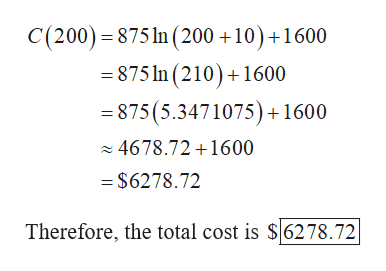# The total cost function for a product isC(x) = 875 ln(x + 10) + 1600where x is the number of units produced.(a) Find the total cost of producing 200 units. (Round your answer to the nearest cent.)\$ (b) Producing how many units will give total costs of \$9500? (Round your answer to the nearest whole number.) units

Question

The total cost function for a product is

C(x) = 875 ln(x + 10) + 1600

where x is the number of units produced.

(a) Find the total cost of producing 200 units. (Round your answer to the nearest cent.)

(b) Producing how many units will give total costs of \$9500? (Round your answer to the nearest whole number.)
units
check_circleExpert Solution
Step 1

The given total cost function for a product is,

Step 2

(a)

Substitute x = 200 in the total cost function and obtain th...help_outlineImage TranscriptioncloseC(200)875 n (200 +10) +1600 875 1n (210)+1600 875 (5.3471075)1600 4678.72 +1600 = \$6278.72 Therefore, the total cost is S6278.72 fullscreen

### Want to see the full answer?

See Solution

#### Want to see this answer and more?

Solutions are written by subject experts who are available 24/7. Questions are typically answered within 1 hour*

See Solution
*Response times may vary by subject and question
Tagged in

### Other